# Analysis of tyrannosaurid growth curves

### Chapter 7: Case studies using hierarchical modelling

We analyze the data from 20 fossils to estimate the growth curves of four tyrannosaurid species: Albertosaurus, Daspletosaurus, Gorgosaurus and Tyrannosaurus. The data are taken from Table 1 of

Erickson, GM et al (2004). Gigantism and comparative life-history parameters of tyrannosaurid dinosaurs. Nature, 430, 772-775.

Let $$Y_{ij}$$ and $$X_{ij}$$ be the body mass and age, respectively, of sample $$i$$ from species $$j=1,…,4$$. We model the data as $Y_{ij} = f_j(X_{ij})\epsilon_{ij}$ where $$f_j$$ is the true growth curve for species $$j$$ and $$\epsilon_{ij}$$ is multiplicative error. We use multiplicative error rather than additive error because variation in the population likely increases with mass/age. Assuming the errors are log-normal the model becomes $\log(Y_{ij}) \sim \mbox{Normal}\left(\log[f_j(X_{ij})]-\sigma^2_j/2,\sigma^2_j\right),$ where $$\sigma_j^2$$ is the error variance for species $$j$$ and is included in the mean so that the multiplicative errors $$\epsilon_{ij}$$ have mean one. In the analysis below we compare four models that vary by the functional form of the growth curves $$f_j$$ (either log-linear or logistic) and how informative about parameters is shared across species (either not pooled or pooled via Gaussian random effects).

## Load and plot the data

 dat         <- read.csv("Erickson.csv")
dat[15:16,] <- dat[16:15,] #order by age
dat

##             Taxon              Spec... Age   Mass
## 1     Gorgosaurus          FMNH PR2211   5  127.0
## 2     Gorgosaurus        RTMP 86.144.1   7  229.0
## 3     Gorgosaurus         RTMP 99.33.1  14  607.0
## 4     Gorgosaurus         RTMP 73.30.1  14  747.0
## 5     Gorgosaurus       RTMP 94.12.602  18 1105.0
## 6   Albertosaurus      RTMP 2002.45.46   2   50.3
## 7   Albertosaurus        RTMP 86.64.01  15  762.0
## 8   Albertosaurus USNM 12814/AMNH 5428  18 1013.0
## 9   Albertosaurus            AMNH 5432  22 1282.0
## 10  Albertosaurus         RTMP 81.10.1  24 1142.0
## 11 Daspletosaurus        RTMP 94.143.1  10  496.0
## 12 Daspletosaurus            AMNH 5438  17 1518.0
## 13 Daspletosaurus          FMNH PR 308  21 1791.0
## 14  Tyrannosaurus           LACM 28471   2   29.9
## 15  Tyrannosaurus           LACM 23845  14 1807.0
## 16  Tyrannosaurus           AMNH 30564  15 1761.0
## 17  Tyrannosaurus        ICM 2001.90.1  16 2984.0
## 18  Tyrannosaurus          RTMP 81.6.1  18 3230.0
## 19  Tyrannosaurus         RTMP 81.12.1  22 5040.0
## 20  Tyrannosaurus         FMNH PR 2081  28 5654.0

 taxon       <- dat[,1]
age         <- dat[,3]
mass        <- dat[,4]

# Plot the data

plot(NA,xlim=range(age),ylim=range(mass),
main="", xlab="Age (years)", ylab="Body Mass (kg)")
points(age[taxon=="Albertosaurus"], mass[taxon=="Albertosaurus"], pch=1,cex=1.5)
points(age[taxon=="Daspletosaurus"],mass[taxon=="Daspletosaurus"],pch=2,cex=1.5)
points(age[taxon=="Gorgosaurus"],   mass[taxon=="Gorgosaurus"],   pch=3,cex=1.5)
points(age[taxon=="Tyrannosaurus"], mass[taxon=="Tyrannosaurus"], pch=4,cex=1.5)

# Fitted growth curves (from the original paper)

x <- seq(0,30,.1)
lines(x,1218/(1+exp(-0.43*(x-14.1)))+5, lty=1)
lines(x,1728/(1+exp(-0.44*(x-12.1)))+5, lty=2)
lines(x,1234/(1+exp(-0.38*(x-12.4)))+5, lty=3)
lines(x,5551/(1+exp(-0.57*(x-16.1)))+5, lty=4)

legend("topleft",c("Albertosaurus","Daspletosaurus","Gorgosaurus","Tyrannosaurus"),
lty=1:4, pch=1:4,bty="n",cex=1.5)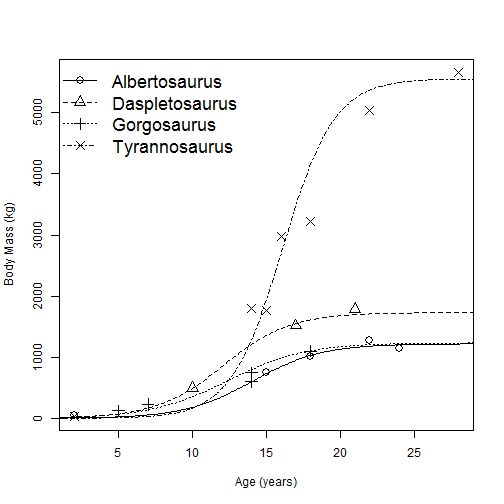## Put the data in JAGS format

 library(rjags)
set.seed(0820)

y       <- log(mass)
x       <- log(age)
n       <- length(y)
sp      <- as.numeric(taxon)
names   <- c("Albertosaurus","Daspletosaurus","Gorgosaurus","Tyrannosaurus")
data    <- list(y=y,x=x,sp=sp,n=n,N=4)
data

## $y ##  4.844187 5.433722 6.408529 6.616065 7.007601 3.918005 6.635947 ##  6.920672 7.156177 7.040536 6.206576 7.325149 7.490529 3.397858 ##  7.499423 7.473637 8.001020 8.080237 8.525161 8.640119 ## ##$x
##   1.6094379 1.9459101 2.6390573 2.6390573 2.8903718 0.6931472 2.7080502
##   2.8903718 3.0910425 3.1780538 2.3025851 2.8332133 3.0445224 0.6931472
##  2.6390573 2.7080502 2.7725887 2.8903718 3.0910425 3.3322045
##
## $sp ##  3 3 3 3 3 1 1 1 1 1 2 2 2 4 4 4 4 4 4 4 ## ##$n
##  20
##
## $N ##  4   burn <- 10000 iters <- 100000 thin <- 10 plot(x,y,pch=sp,cex=1.5,xlab="Log Age (Years)",ylab="Log Body Mass (kg)") for(j in 1:4){ b <- lm(y[sp==j]~x[sp==j]) abline(b,b,lty=j) } legend("topleft",names,lty=1:4, pch=1:4,bty="n",cex=1.5)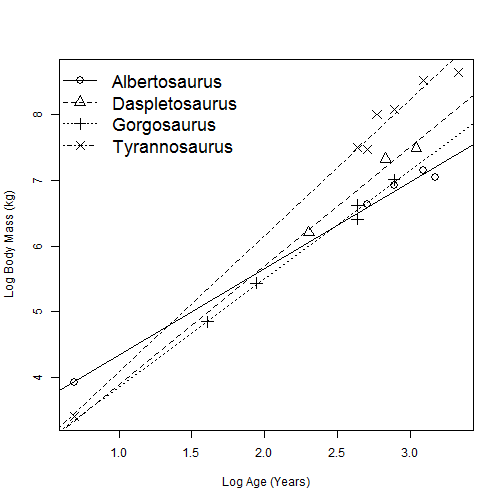Summary: The log of age versus the log of mass is roughly linear for all four taxa. ## Model 1: Unpooled analysis with log-linear growth curves The exponential growth model is $f_{j}(age) = A_{j}age^{b_{j}} \mbox{ so that } \log[f_{j}(age)] = a_{j}+b_{j}\log(age),$ where $$log(A_j) = a_j$$. This is clearly unrealistic when applied to the entire life-span of a species because body mass should plateau, but may be reasonable to model growth early in life. In this analysis the priors are $$a_j,b_j\sim\mbox{Normal}(0,10)$$ and $$\sigma^2_j\sim\mbox{InvGamma}(0.1,0.1)$$ to give a separate regression for each species.  model_string1 <- textConnection("model{ for(i in 1:n){ y[i] ~ dnorm(muY[i],tau[sp[i]]) muY[i] <- a[sp[i]] + b[sp[i]]*x[i] - 0.5/tau[sp[i]] } for(j in 1:N){ a[j] ~ dnorm(0.0,0.1) b[j] ~ dnorm(0.0,0.1) tau[j] ~ dgamma(0.1,0.1) } for(age in 1:30){for(j in 1:4){ fitted[age,j] <- exp(a[j] + b[j]*log(age)) }} }") model1 <- jags.model(model_string1,data = data, quiet=TRUE,n.chains=2) update(model1, burn, progress.bar="none") samples1 <- coda.samples(model1, variable.names=c("a","b","fitted","tau"), n.iter=iters, thin=thin, progress.bar="none") ESS <- effectiveSize(samples1) ESS[which.min(ESS)]  ## a ## 3922.673   ESS[which.max(ESS)]  ## fitted[9,3] ## 20000   fit1 <- summary(samples1)$quantiles[1:120+8,3]
lo1  <- summary(samples1)$quantiles[1:120+8,1] hi1 <- summary(samples1)$quantiles[1:120+8,5]
id   <- rep(1:4,each=30)

par(mfrow=c(2,2))
for(j in 1:4){
plot(NA,xlim=range(age),ylim=range(mass),
xlab="Age (years)", ylab="Body Mass (kg)",
main=names[j])
points(age[sp==j],mass[sp==j],pch=19,cex=1.5)
lines(1:30,fit1[id==j],lty=1)
lines(1:30,lo1[id==j],lty=2)
lines(1:30,hi1[id==j],lty=2)

if(j==1){
legend("topleft",c("Data","Post mean","95% interval"),
pch=c(19,NA,NA),lty=c(NA,1,2),cex=1.5,bty="n")
}
}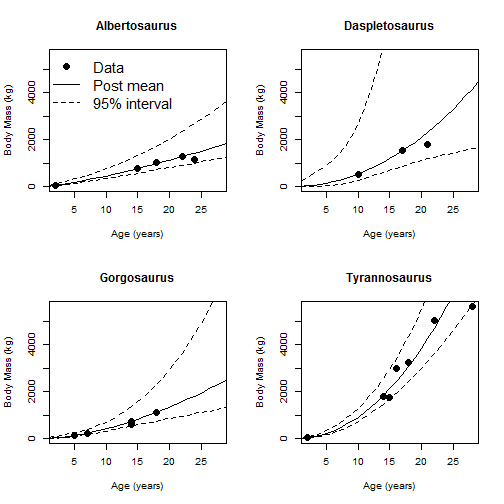Summary: Convergence is OK (large ESS) and the fit to the data is decent. However, the uncertainty in the mean growth curves is very large.

## Model 2: Pooled analysis with log-linear growth curves

This model is the same as Model 1 except that all species have the same variance, $$\sigma^2_j=\sigma^2$$, and the regression coefficients are modeled hierarchically. For example, for intercepts we have $$a_j\sim\mbox{Normal}(\mu_a,\sigma_a^2)$$, where $$\mu_a\sim\mbox{Normal}(0,10)$$ and $$\sigma^2_a\sim\mbox{InvGamma}(0.1,0.1)$$. The slopes $$b_j$$ are modelled similarly.

model_string2 <- textConnection("model{
for(i in 1:n){
y[i]    ~ dnorm(muY[i],tau)
muY[i] <- a[sp[i]] + b[sp[i]]*x[i]  - 0.5/tau
}

for(j in 1:N){
a[j]   ~ dnorm(mu,tau)
b[j]   ~ dnorm(mu,tau)
}

for(k in 1:2){
mu[k]  ~ dnorm(0.0,0.1)
}
for(k in 1:3){
tau[k]  ~ dgamma(0.1,0.1)
}

for(age in 1:30){for(j in 1:4){
fitted[age,j] <- exp(a[j] + b[j]*log(age))
}}
}")

model2   <- jags.model(model_string2,data = data,quiet=TRUE, n.chains=2)
update(model2, burn, progress.bar="none")
samples2 <- coda.samples(model2, variable.names=c("a","b","fitted","tau"), n.iter=iters, thin=thin, progress.bar="none")

ESS <- effectiveSize(samples2)
ESS[which.min(ESS)]

##    b
## 4140.49

 ESS[which.max(ESS)]

## fitted[17,4]
##     20723.92

 fit2 <- summary(samples2)$quantiles[1:120+8,3] lo2 <- summary(samples2)$quantiles[1:120+8,1]
hi2  <- summary(samples2)$quantiles[1:120+8,5] id <- rep(1:4,each=30) par(mfrow=c(2,2)) for(j in 1:4){ plot(NA,xlim=range(age),ylim=range(mass), xlab="Age (years)", ylab="Body Mass (kg)", main=names[j]) points(age[sp==j],mass[sp==j],pch=19,cex=1.5) lines(1:30,fit2[id==j],lty=1) lines(1:30,lo2[id==j],lty=2) lines(1:30,hi2[id==j],lty=2) if(j==1){ legend("topleft",c("Data","Post mean","95% interval"), pch=c(19,NA,NA),lty=c(NA,1,2),cex=1.5,bty="n") } }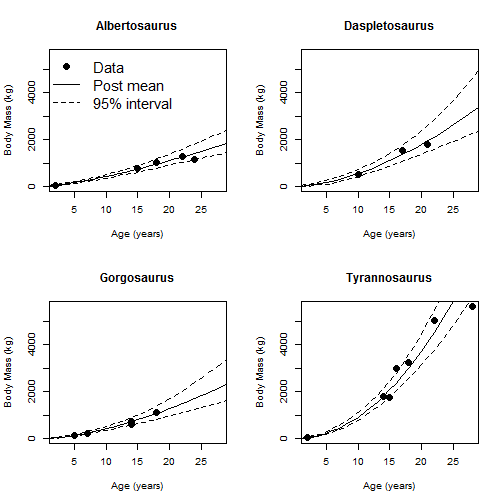Summary: The posterior mean growth curve is similar to Model 1, but the posterior variance is small; this is the effect of pooling information across curves. ## Model 3: Non-pooled analysis with logistic growth curves The non-linear model is ($$x = \log$$(age)) $f_j(x) = a_{j}+b_{j}\frac{1}{1+exp[-(x-c_{j})/d_j]},$ which in an increasing function that plateaus as age increases. The growth curve for species $$j$$ determined by four parameters: 1. $$a_j$$ is the average mass at age 0 2. $$b_j$$ is the lifetime expected gain in mass 3. $$\log(c_j)$$ is the age at which the species is half way to maturity 4. $$d_j$$ determines the rate of increase For the curve to be positive and increasing for all ages, we must have $$a_j>0$$, $$b_j>a_j$$ and $$d_j>0$$. We enforce these constraints by representing the growth-curve parameters in terms of unconstrained parameters $$\alpha_{j1},…,\alpha_{j4}$$ as: 1. $$a_j=\exp(\alpha_{j1})$$ 2. $$b_j=\exp(\alpha_{j2})$$ 3. $$c_j=\alpha_{j3}$$ 4. $$d_j=\exp(\alpha_{j4})$$ In this analysis we use uninformative prior for all parameters, separately by species: $$\alpha_{jk}\sim\mbox{Normal}(0,10)$$ and $$\sigma^2_j\sim\mbox{InvGamma}(0.1,0.1)$$. Note that for any value of the $$\alpha_{jk}$$, the growth curve is positive and increasing for all ages.  model_string3 <- textConnection("model{ for(i in 1:n){ y[i] ~ dnorm(muY[i],tau[sp[i]]) muY[i] <- log(a[sp[i]] + b[sp[i]]/(1+exp(-part[i]))) - 0.5/tau[sp[i]] part[i] <- (x[i]-c[sp[i]])/d[sp[i]] } for(j in 1:N){ a[j] <- exp(alpha[j,1]) b[j] <- exp(alpha[j,2]) c[j] <- alpha[j,3] d[j] <- exp(alpha[j,4]) for(k in 1:4){alpha[j,k] ~ dnorm(0.0,0.1)} tau[j] ~ dgamma(0.1,0.1) } for(age in 1:30){for(j in 1:4){ PART[age,j] <- (log(age)-c[j])/d[j] fitted[age,j] <- a[j] + b[j]/(1+exp(-PART[age,j])) }} }") model3 <- jags.model(model_string3,data = data,quiet=TRUE, n.chains=2) update(model3, burn, progress.bar="none") samples3 <- coda.samples(model3, variable.names=c("a","b","c","d","fitted","tau"), n.iter=iters, thin=thin, progress.bar="none") ESS <- effectiveSize(samples3) ESS[which.min(ESS)]  ## c ## 1232.332   ESS[which.max(ESS)]  ## fitted[14,3] ## 20000   fit3 <- summary(samples3)$quantiles[1:120+16,3]
lo3  <- summary(samples3)$quantiles[1:120+16,1] hi3 <- summary(samples3)$quantiles[1:120+16,5]
id   <- rep(1:4,each=30)

par(mfrow=c(2,2))
for(j in 1:4){
plot(NA,xlim=range(age),ylim=range(mass),
xlab="Age (years)", ylab="Body Mass (kg)",
main=names[j])
points(age[sp==j],mass[sp==j],pch=19,cex=1.5)
lines(1:30,fit3[id==j],lty=1)
lines(1:30,lo3[id==j],lty=2)
lines(1:30,hi3[id==j],lty=2)

if(j==1){
legend("topleft",c("Data","Post mean","95% interval"),
pch=c(19,NA,NA),lty=c(NA,1,2),cex=1.5,bty="n")
}
}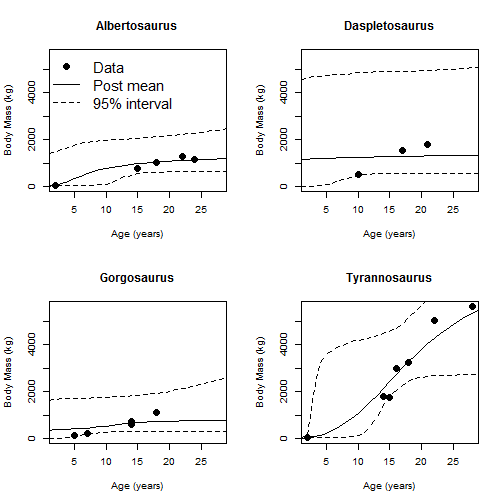Summary: Convergence is questionable (low ESS) and the 95% intervals for the curves are very wide. I appears that there is not enough data to estimate a non-linear growth curve separately by species.

## Model 4: Pooled analysis of logistic growth curves

This model is the same as Model 3 except that all species have the same variance, $$\sigma^2_j=\sigma^2$$, and the regression coefficients are modeled hierarchically. The random effect distributions are $$\log(\alpha_{jk})\sim\mbox{Normal}(\mu_k,\sigma_k^2)$$, where $$\mu_k\sim\mbox{Normal}(0,10)$$ and $$\sigma^2_k\sim\mbox{InvGamma}(0.1,0.1)$$.

 model_string4 <- textConnection("model{
for(i in 1:n){
y[i]    ~ dnorm(muY[i],tau)
muY[i]  <- log(a[sp[i]] + b[sp[i]]/(1+exp(-part[i])))  - 0.5/tau
part[i] <- (x[i]-c[sp[i]])/d[sp[i]]
}

for(j in 1:N){
a[j]   <- exp(alpha[j,1])
b[j]   <- exp(alpha[j,2])
c[j]   <- alpha[j,3]
d[j]   <- exp(alpha[j,4])

for(k in 1:4){alpha[j,k]   ~ dnorm(mu[k],tau[k])}
}

for(j in 1:4){
mu[j]  ~ dnorm(0,0.1)
tau[j] ~ dgamma(0.1,0.1)
}
tau ~ dgamma(0.1,0.1)

for(age in 1:30){for(j in 1:4){
PART[age,j]   <- (log(age)-c[j])/d[j]
fitted[age,j] <- a[j] + b[j]/(1+exp(-PART[age,j]))
}}

}")

model4   <- jags.model(model_string4,data = data,quiet=TRUE, n.chains=2)
update(model4, burn, progress.bar="none")

samples4 <- coda.samples(model4, variable.names=c("a","b","c","d","fitted","tau"), n.iter=iters, thin=thin, progress.bar="none")

ESS <- effectiveSize(samples4)
ESS[which.min(ESS)]

##     c
## 303.9109

 ESS[which.max(ESS)]

## fitted[2,1]
##    19001.98

 fit4 <- summary(samples4)$quantiles[1:120+16,3] lo4 <- summary(samples4)$quantiles[1:120+16,1]
hi4  <- summary(samples4)\$quantiles[1:120+16,5]
id   <- rep(1:4,each=30)

par(mfrow=c(2,2))
for(j in 1:4){
plot(NA,xlim=range(age),ylim=range(mass),
xlab="Age (years)", ylab="Body Mass (kg)",
main=names[j])
points(age[sp==j],mass[sp==j],pch=19,cex=1.5)
lines(1:30,fit4[id==j],lty=1)
lines(1:30,lo4[id==j],lty=2)
lines(1:30,hi4[id==j],lty=2)

if(j==1){
legend("topleft",c("Data","Post mean","95% interval"),
pch=c(19,NA,NA),lty=c(NA,1,2),cex=1.5,bty="n")
}
}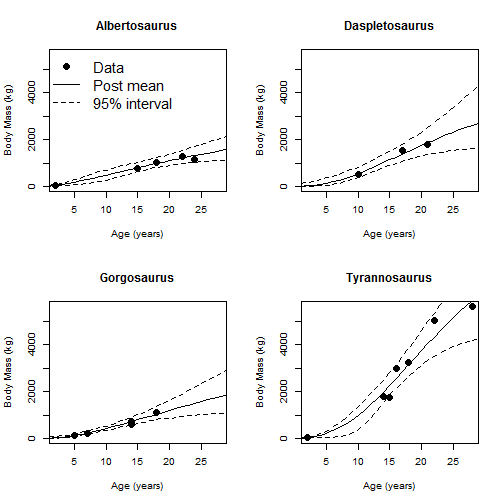Summary: Compared to Model 3, this fit has better convergence and much more narrow 95% intervals. By sharing information across curves we are able to estimate non-linear means for each species.

## Model comparison

 # No pooling, log-linear model
dic1 <- dic.samples(model1, n.iter=iters, thin=thin, progress.bar="none")
dic1

## Mean deviance:  3.496
## penalty 25
## Penalized deviance: 28.5

 # Pooling, log-linear model
dic2 <- dic.samples(model2, n.iter=iters, thin=thin, progress.bar="none")
dic2

## Mean deviance:  -12.54
## penalty 9.154
## Penalized deviance: -3.386

 # No pooling, logistic model
dic3 <- dic.samples(model3, n.iter=iters, thin=thin, progress.bar="none")
dic3

## Mean deviance:  22.67
## penalty 41.59
## Penalized deviance: 64.26

 # Pooling, logistic model
dic4 <- dic.samples(model4, n.iter=iters, thin=thin, progress.bar="none")
dic4

## Mean deviance:  -14.02
## penalty 12.2
## Penalized deviance: -1.828


Summary: Both hierarchical models that borrow information across taxa have much smaller DIC than models that analyze taxa separately. The log-linear model edges out the logistic model for the smallest DIC (best model).